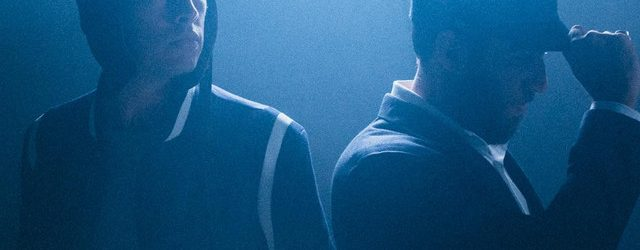# Claire de Lune

## Flight Facilities## Claire de Lune testo

If I would know you, would you know me,
If I would know you, would you know me,
If I would know you, would you know me,
If I would know you, would you know me,

Don’t go, Tell me that the lights won’t change,
Tell me that you’ll feel the same, And we’ll stay here forever,
Don’t go, Tell me that the lights won’t change,
Tell me that it’ll stay the same, Where we go,
Where we,
Where we go, Where we go,
Where we go, Where we go, Where we go.

Where we go, Where we,
Where we go, Where we,
Where we go, Where we,
Where we go, Where we go

Where we go, Where we,
Where we go, Where we,
Where we go, Where we,
Where we go, Where we go.

Where we go, Where we,
Where we go, Where we,
Where we go, Where we,
Where we go, Where we go.

Where we go, Where we,
Where we go, Where we,
Where we go, Where we,
Where we go, Where we go.

Don’t go, Tell me that the lights won’t change,
Tell me that you’ll feel the same, And we’ll stay here forever,
Don’t go, Tell me that the lights won’t change,
Tell me that it’ll stay the same, Where we go,
Where we,
Where we go, Where we go,
Where we go, Where we go, Where we go.

If I would know you, would you know me,
If I would know you, would you know me,
If I would know you, would you know me,
If I would know you, would you know me,

Don’t go, Tell me that the lights won’t change,
Tell me that we’ll stay the same, Where we go,
Where we,
Where we go, Where we go,
Where we go, Where we go, Where we go.